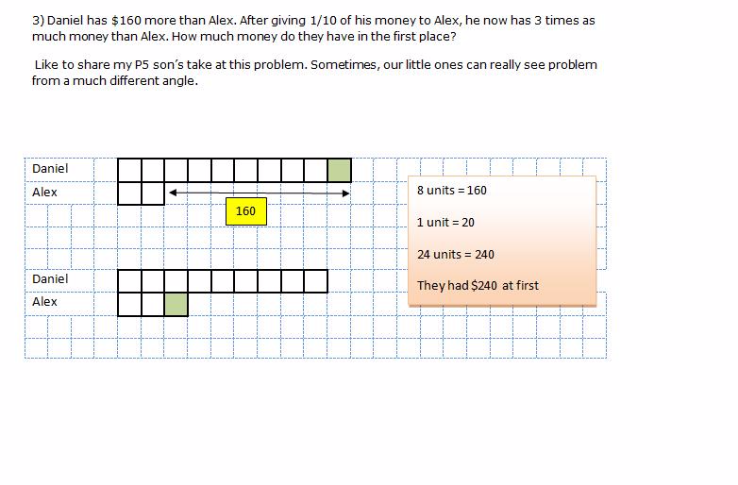# Question

Daniel has \$160 more than Alex. After giving 1/10 of his money to Alex, he now has 3 times as much money than Alex. How much money do they have in the first place?

After —
Daniel : 9 units
Alex : 3 units

At first —
Daniel : 10 units
Alex : 2 units

10 units – 2 units = 8 units = 160
1 unit = 20
9 units + 3 units = 12 units = 12 x 20 = 240

Ans : \$240.

0 Replies 0 Likes

at beginning, Daniel has 10u
after Daniel give Alex 1 u,
now, Daniel has 9u, and Alex has 3 u ( Daniel has 3 times more than Alex)
so at the beginning, Alex has 2u, so 10u – 2u = 160, 1u is 20

0 Replies 1 Like

D-A=160

A = D-160

(9/10)D = 3(A+[1/10]D)

(9/10)D = 3([D-160]+[1/10]D)

(9/10)D = 3D – 480 + [3/10] D

480 = 12/5 D

D = 200

A = 200-160 = 40

0 Replies 0 Likes

1)  d = a + 160

2) 0.9d = (a + 0.1d) * 3

d = 5a

4a = 160

a = 40

d =200

0 Replies 0 Likes

Before :
Daniel : 10 units + \$160
Alex : 10 units

After :
Daniel : 3 parts
Alex : 1 part

Since Daniel gives 1/10 to Alex, he has given (1 unit + \$16) to Alex

In the “After” model,

1 part (for Alex) = 11 units + \$16
3 parts (for Daniel) = 9 units + \$144 ; ie. 1 part for Daniel is 3 units + \$48

The difference is 2 parts : this is the key

2(11units + 16) = 6 units + \$96
22 units + \$32 = 6 units + \$96
16 units = \$64
1 unit = \$4

At first,
Alex has 10 units = 10 X \$4 = \$40
Daniel has 10 units + \$160 = \$40 + \$160 = \$200.

0 Replies 0 Likes0 Replies 0 Likes

Not sure how to draw model here. Need to have before and after model.

Before :
Daniel : 10 units + \$160
Alex : 10 units

After :
Daniel : 3 parts
Alex : 1 part

Since Daniel gives 1/10 to Alex, he has given (1 unit + \$16) to Alex

In the “After” model,

1 part (for Alex) = 11 units + \$16
3 parts (for Daniel) = 9 units + \$144 ; ie. 1 part for Daniel is 3 units + \$48

The difference is 2 parts : this is the key

2(11units + 16) = 6 units + \$96
22 units + \$32 = 6 units + \$96
16 units = \$64
1 unit = \$4

At first,
Alex has 10 units = 10 X \$4 = \$40
Daniel has 10 units + \$160 = \$40 + \$160 = \$200.

0 Replies 1 Like